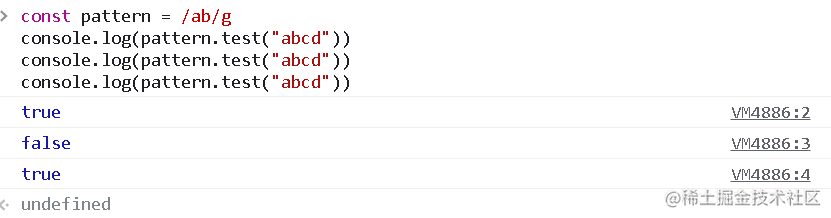# 小心！正则 test() 匹配的一个“坑”``````const pattern = /ab/g
pattern.test("abcd") // true
``````

``````const pattern = /ab/g
console.log(pattern.test("abcd"))
console.log(pattern.test("abcd"))
console.log(pattern.test("abcd"))
``````

“不就是三个 true 吗？！”lastIndex 属性用于规定下次匹配的起始位置。

``````const pattern = /ab/g
console.log(pattern.test("abcd")) // true
console.log(pattern.lastIndex) // 2
console.log(pattern.test("abcd")) // false
console.log(pattern.lastIndex) // 0
console.log(pattern.test("abcd")) // true
console.log(pattern.lastIndex) // 2
````````````const pattern = /ab/g
console.log(pattern.test("abcd")) // true
pattern.lastIndex = 0
console.log(pattern.test("abcd")) // true
``````

``````const pattern = /ab/g
console.log("abcd".match(pattern)) // ['ab']
console.log("abcdab".match(pattern)) // ['ab', 'ab']
console.log("123".match(pattern)) // null
``````

match 是匹配的更优解，不用手动清理，存在则悉数返回成数组，不存在，返回 nullOK，以上便是本篇分享。点赞关注评论，为好文助力👍